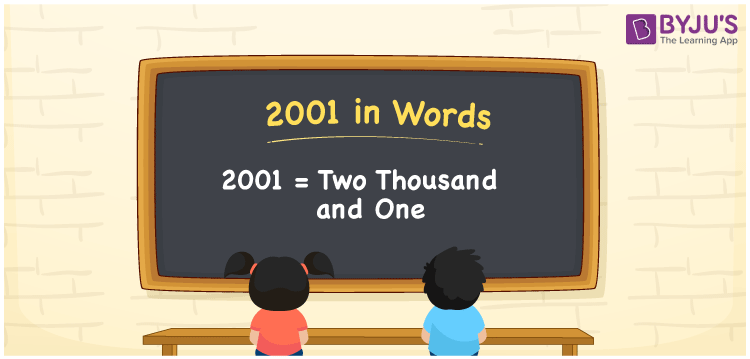# 2001 in Words

In Mathematics, the number 2001 is a cardinal number. The number 2001 in words is “Two Thousand and One”. To convert 2001 into words, we will use the place value system. For example, Now, let us discuss the spelling of 2001 in English words and the procedure of writing the number name 2001 in detail.

 2001 in Words: Two Thousand and One. Two Thousand and One in Numerical Form: 2001.

## 2001 in English Words## How to Write 2001 in Words?

The following table presents the place values of the four-digit number 2001:

 Thousands Hundreds Tens Ones 2 0 0 1

The expanded form of 2001 is as follows:

= 2 × Thousand + 0 × Hundred + 0 × Ten + 1 × One

= 2 × 1000 + 0 × 100 + 0 × 10 + 1 × 1

= 2000 + 1

= 2001

= Two thousand and one

Hence, 2001 in words is two thousand and one.

The number 2001 is a natural number that appears after 2000 but before 2002.

2001 in words – Two thousand and one

Is 2001 an odd number? – Yes

Is 2001 an even number? – No

Is 2001 a perfect square number? – No

Is 2001 a perfect cube number? – No

Is 2001 a prime number? – No

Is 2001 a composite number? – Yes

## Frequently Asked Questions on 2001 in Words

Q1

### Write 2001 in words.

2001 in words is two thousand and one.

Q2

### Simplify 2000 + 1, and express it in words.

Simplifying 2000 + 1, we get 2001. Hence, 2001 in words is two thousand and one.

Q3

### Is 2001 an odd number?

Yes, 2001 is an odd number.